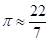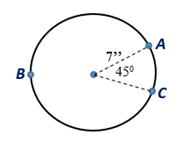Chapter 8.CT, Problem 11CTElementary Geometry For College St...

7th Edition
Alexander + 2 others
ISBN: 9781337614085

Solutions

Chapter
SectionElementary Geometry For College St...

7th Edition
Alexander + 2 others
ISBN: 9781337614085
Textbook Problem

Where, find the approximate length of A C ^ .To determine

To find:

The approximate length of AC^.

Explanation

Given:

If and the figure given below.

Theorem:

1. The circumference C of a circle is given by the formula, C=πd or C=2πr where d is the diameter and r is the radius of the circle.

2. In a circle whose circumference is C, the length l of an arc whose degree measure is m is given by l=m360·C.

From the given, .

Form the figure, m=45, and r=7 in.

To find the circumference of the circle, substitute r=7 in C=2πr

Still sussing out bartleby?

Check out a sample textbook solution.

See a sample solution

The Solution to Your Study Problems

Bartleby provides explanations to thousands of textbook problems written by our experts, many with advanced degrees!

Get Started